Solving Multi-Step Linear Inequalities (single variable) MathBitsNotebook.com Terms of Use   Contact Person: Donna RobertsFor more introductory information see Solving Two Step Linear Inequalities.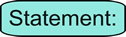The process for solving a linear inequality is the same as that for solving a linear equation, with one important exception ...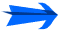... when you multiply (or divide) an inequality by a negative value, you must change the direction of the inequality.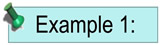Solve and graph the solution set of:   5(x - 3) > 10 Proceed as you would to solve a linear equation. Distribute (remove the parentheses). Add 15 to both sides. Divide both sides by 5. Note: The direction of the inequality stays the same since we did NOT multiply by a negative value. Graph using a open circle for 5 (since x can NOT equal 5) and an arrow to the right (since our symbol is greater than).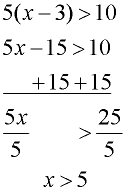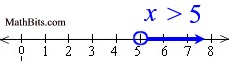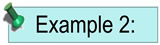Solve and graph the solution set of: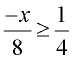Multiply both sides by the least common denominator, which is 8. Divide both sides by -1, and flip the direction of the inequality. Note: The direction of the inequality was reversed since we divided by a negative value (-1). Graph using a closed circle for -2 (since x can equal -2) and an arrow to the left (since our symbol is less than or equal to).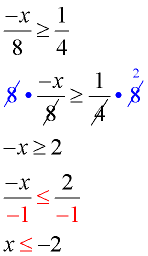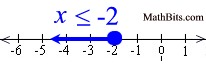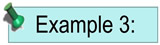Solve and graph the solution set of: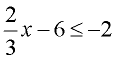Add 6 to both sides. Multiply both sides by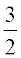. Note: The direction of the inequality stays the same since we did NOT multiply by a negative value. Graph using a closed circle for 6 (since x can equal 6) and an arrow to the left (since our symbol is less than or equal to).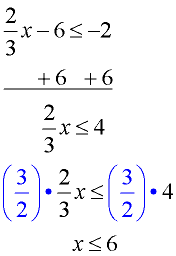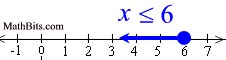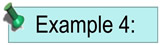Solve and graph the solution set of: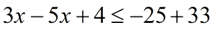Combine the x-values on the left side. Combine the numeric values on the right. Subtract 4 from both sides. Divide both sides by -2. Note: The direction of the inequality was reversed since we divided by a negative value. Graph using a closed circle for -2 (since x can equal -2).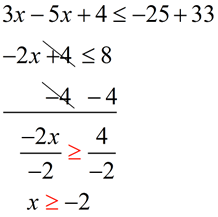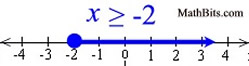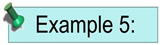Solve and graph the solution set of:   5 - 2x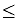13 + 2x Add 2x to both sides. Subtract 13 from both sides. Divide both sides by 4. Note: There was no multiplication or division by a negative value, so the inequality symbol did not get reversed. Graph using a closed circle for -2 (since x can equal -2).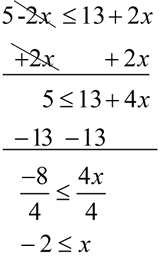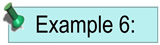Solve and graph the solution set of:   4x + 10 < 3(2x + 4) Distribute across parentheses. Subtract 4x from both sides. Add -12 to both sides. Divide both sides by 2. Note: There was no multiplication or division by a negative value, so the inequality symbol did not get reversed. Graph using a closed circle for -2 (since x can equal -2).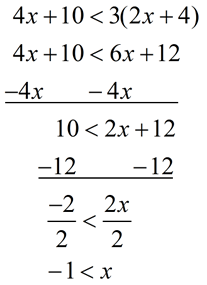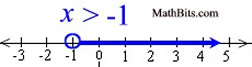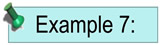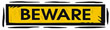Solve and graph the solution set of:    4(2x + 1) < 8x + 4 Distribute across the parentheses. Subtract 8x from both sides. Notice the FALSE result. The solution to this inequality is the "empty set" (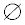). There are no x-values which will make this inequality true. There is nothing to be graphed.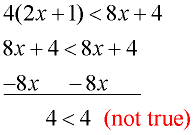This tells us that NO x-values will make this inequality true.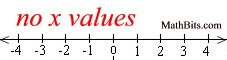In this problem, the left side of the inequality is simply another way of writing the right side of the inequality. The two sides are EQUAL to one another. Since a quantity can never be less than itself, this inequality is never true.Yes, there is a way to determine solutions for inequalities on your graphing calculator. Click the calculator at the right to see how to use the calculator with single variable inequalities.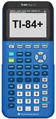For calculator help with inequalities (single variable) click here.